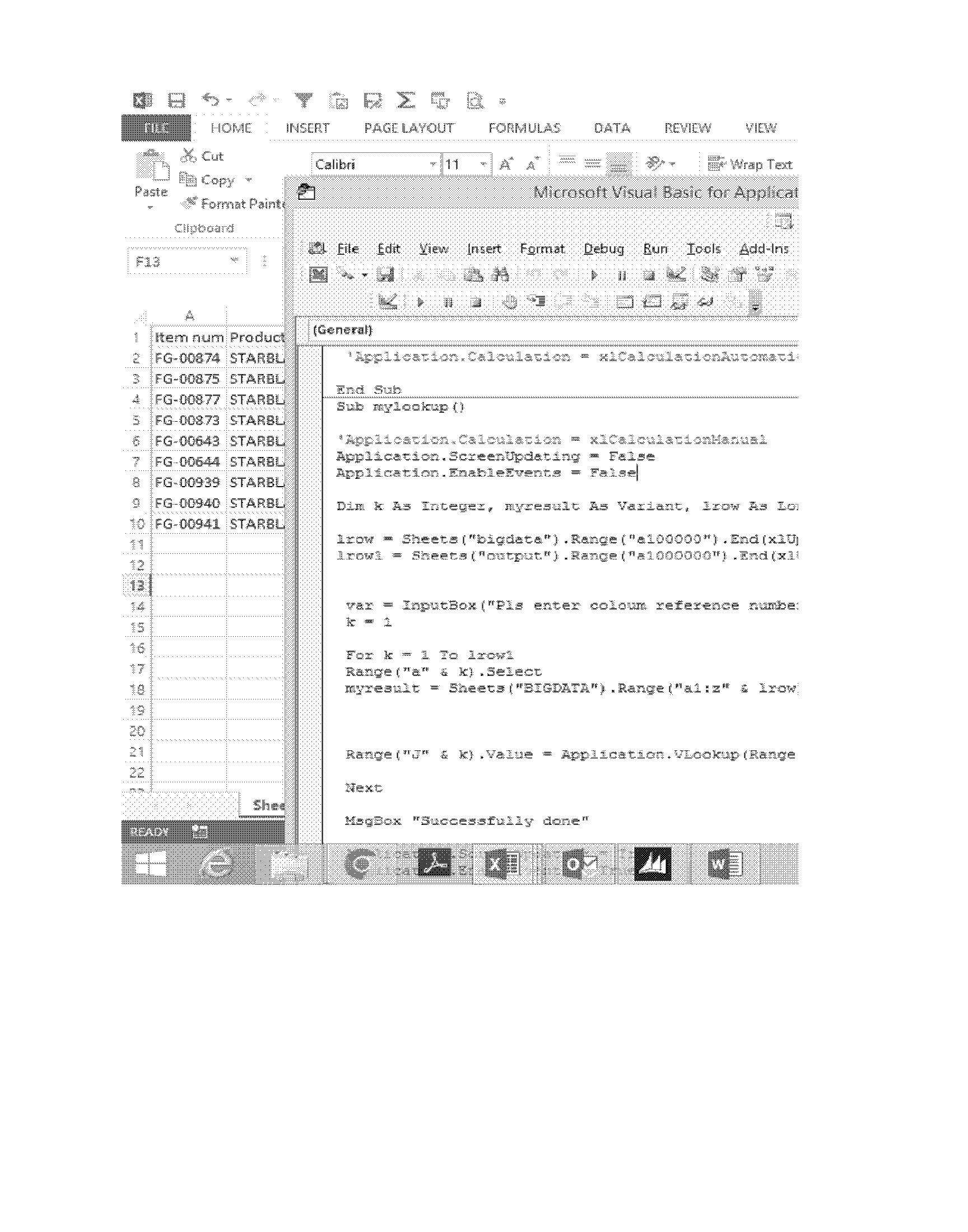# Excel VBA , VBA code for Vlookup, VLOOKUP CODE

Educations 8 Tue 21 Jun 2022Code for excel vba

I have 2 sheets and I rename both sheet with bigdata and output

Bigdata –  this is master file here all data store

Output = where I get lookup value.

1st I have create sub function and used name mylookup ( you can use as per your choice)

Sub mylookup()

Here mention code

End sub

Taken variable here I have taken Dim k As Integer, myresult As Variant, lrow As Long, var As Long, lrow1 As Long

After that I have count both sheets row with dynamically

lrow = Sheets("bigdata").Range("a100000").End(xlUp).Row

lrow1 = Sheets("output").Range("a1000000").End(xlUp).Row

also I have get Coolum reference number means you have looking in which Coolum data .

var = InputBox("Pls enter Coolum reference number")

Variable k used for loop function mean and row reference

Myresult is master data file table name

myresult = Sheets("BIGDATA").Range("a1:z" & lrow)

vlookup code is as below and getting value in Range("J" & k).Value

Range("J" & k).Value = Application.VLookup(Range("a" & k), myresult, var, 0)

ALL CODE

Sub mylookup()

'Application.Calcula­tion = xlCalculation­Manual

Application.ScreenUp­dating = False

Application.EnableEv­ents = False

Dim k As Integer, my­result As Variant, lrow As Long, var As Long, lrow1 As Long

lrow = Sheets("bigda­ta").Range("a100000"­).End(xlUp).Row

lrow1 = Sheets("outp­ut").Range("a1000000­").End(xlUp).Row

var = InputBox("Pls enter coloum refer­ence number")

k = 1

For k = 1 To lrow1

Range("a" & k).Sel­ect

myresult = Sheets(­"BIGDATA").Range("a1­:z" & lrow)

Range("J" & k).Val­ue = Application.VLo­okup(Range("a" & k), myresult, var, 0)

Next

MsgBox "Successful­ly done"

Application.Screen­Updating = True

Application.Enable­Events = True

Application.Calcu­lation = xlCalculati­onAutomatic

End Sub

--Posted By : santosh